# Fractions ProFraction calculator with parentheses and exponents

Intuitive, powerful and easy to use

Looking for an easy to use yet powerful calculator to deal with everyday fraction problems? Fractions Pro is a well designed all-in-one multifunctional calculator to perform calculations with proper and improper fractions, mixed numbers, decimals and integers.

While Fraction Pro can do everything you'd expect from a calculator app, its main focus is fractions, so it lets you perform fraction calculations of any complexity. This unique calculator solves fraction problems on the fly and has a ton of uses.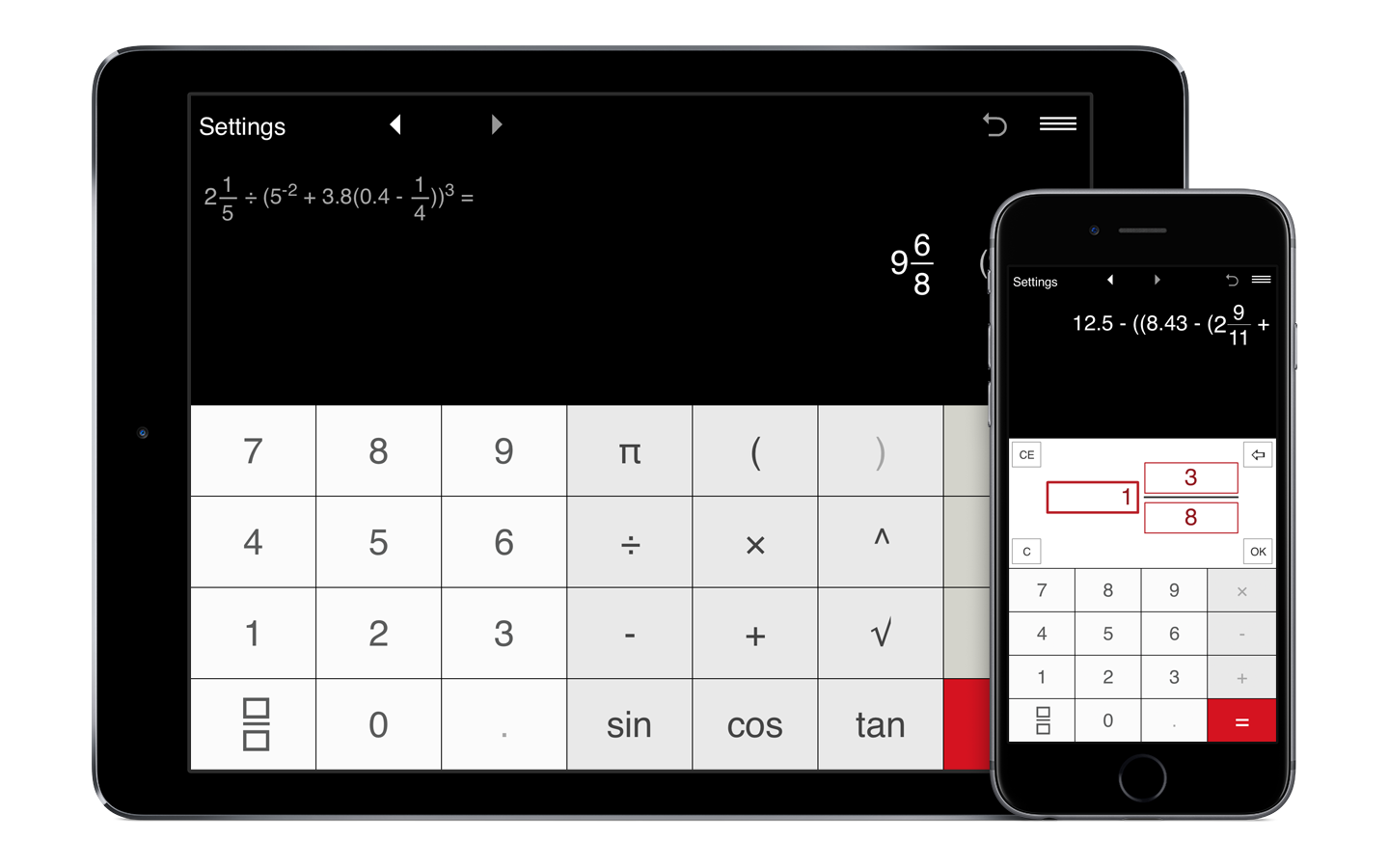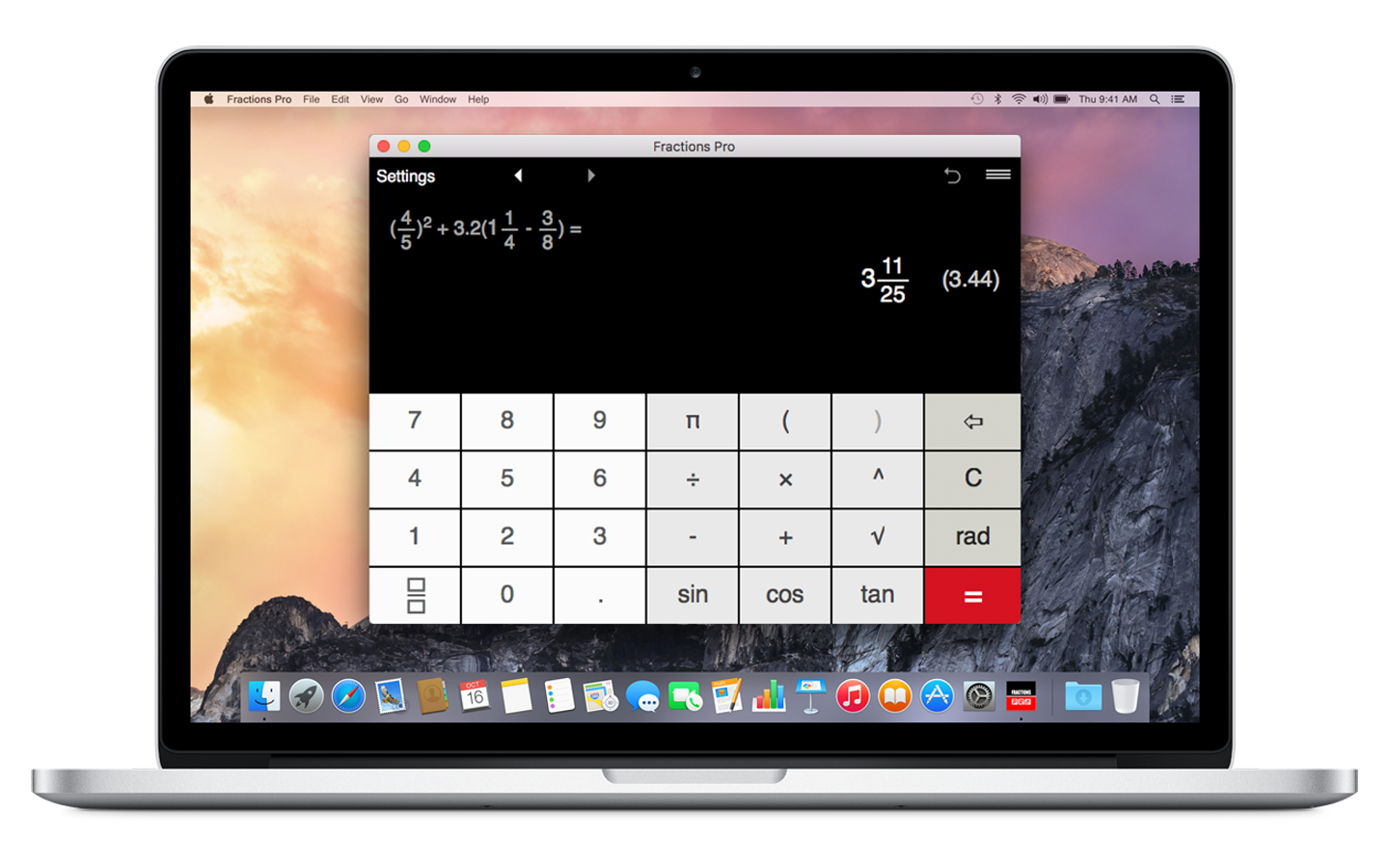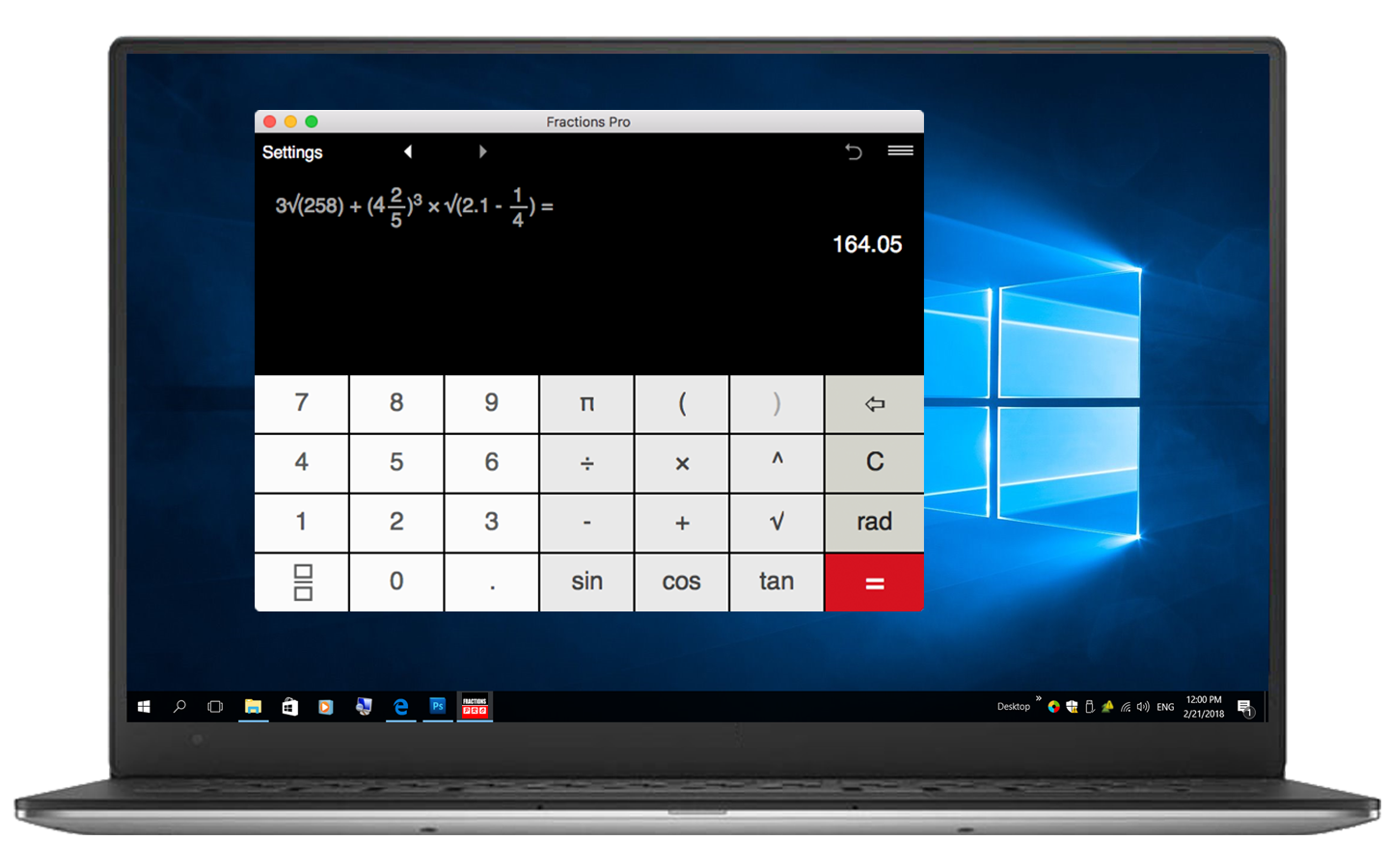Whether you perform engineering or construction calculations involving fractions, check homework, cook or just convert fractions, Fractions Pro is a smart solution for fraction conversion and calculation that saves your time and helps you avoid calculation mistakes.

## Fraction converter and calculator for your smartphone, tablet and computer

### More than fraction calculator and converter

Fractions Pro combines clean intuitive design and lots of really useful options that go beyond what you normally find in fraction calculators and converters.

### Smart and intuitive

The app provides simple input for fraction/decimal math, supports a natural display for fractions and shows both the expression and the result.

### Fraction calculations of any complexity

Ideal tool for engineers, builders, architects, carpenters, designers, scientists, students and anyone who needs to perform fraction calculations and fraction to decimal and decimal to fraction conversion.

### Customize the app to your needs

With Fractions Pro, you may easily get the answer in fractional and decimal forms, round results to usable fractions, select the number of decimal places to display and more.

## Fraction calculator

• Calculator offers intuitive fraction input.
• Complex calculations with fractions, mixed numbers and decimals.
• You may get the answer in a fractional and decimal form.
• For more convenience the app shows both, the expression and the result, at the same time.
• Unlike many fraction calculators, Fraction Pro allows you to round the result to useful fraction.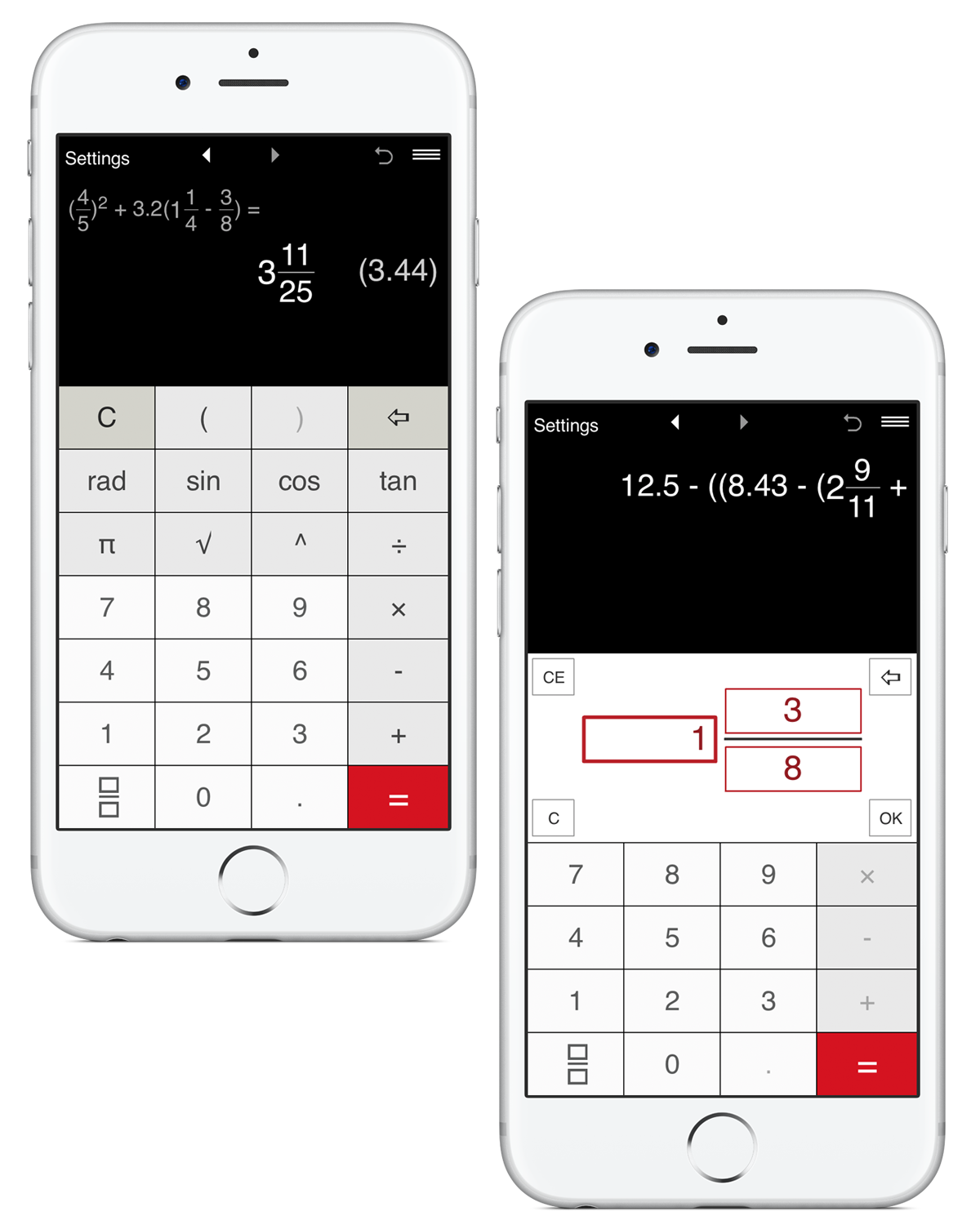## Professional fraction conversion

• Convert between fractions and decimals:
- decimal to fraction conversion
- fraction to decimal conversion
• Simplify fractions and mixed numbers.
• Round the result to the nearest 1/2, 1/4, 1/8, 1/12, 1/16, 1/32, 1/64, 1/128, 1/256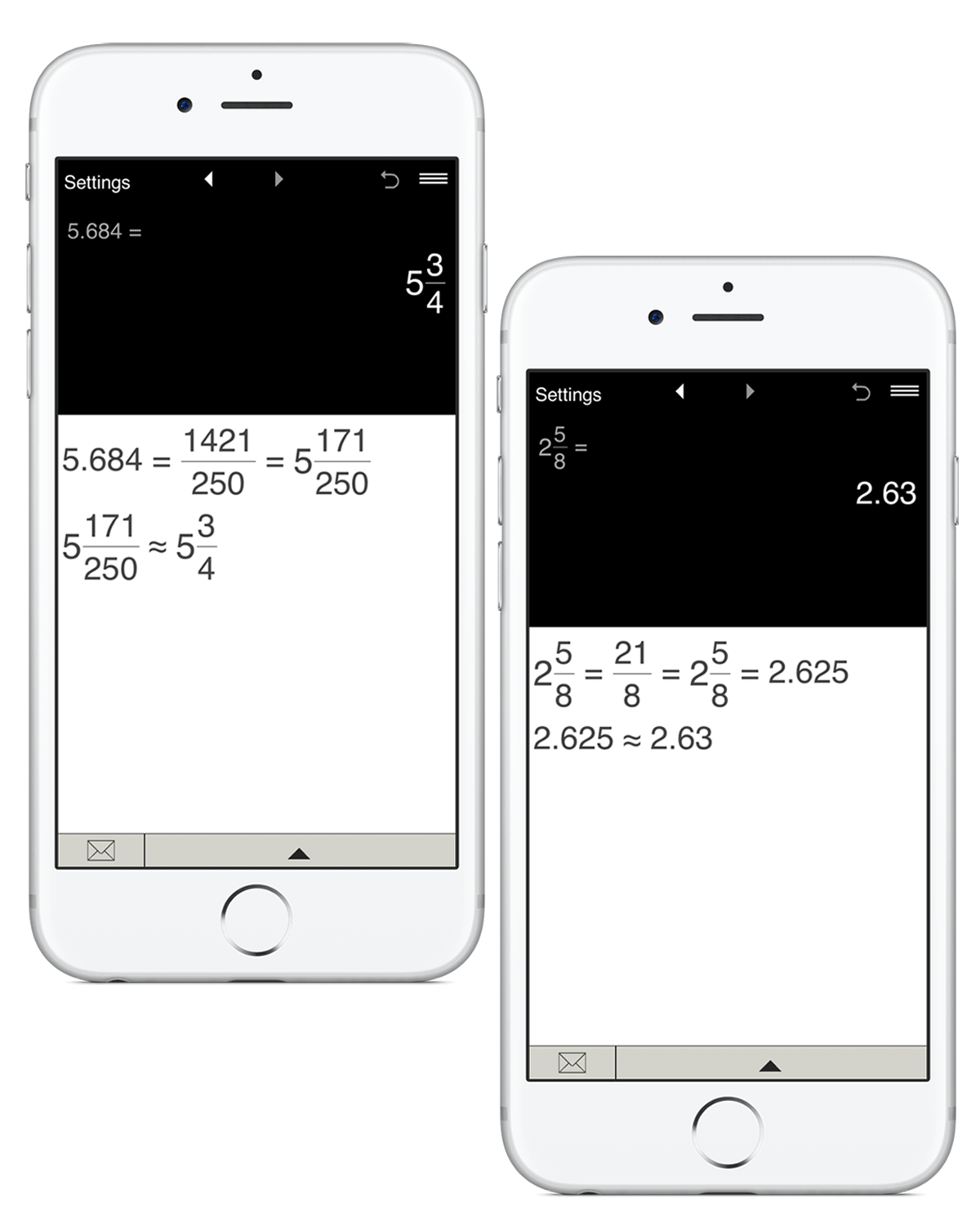## Works as a standard calculator

• Ideal solution for fraction and decimal math.
• Calculations with parentheses, including nested parentheses.
• The app supports the square root, powers, and trigonometric functions.
• Trigonometric calculations in Degrees and Radians.
• Constants: π (pi).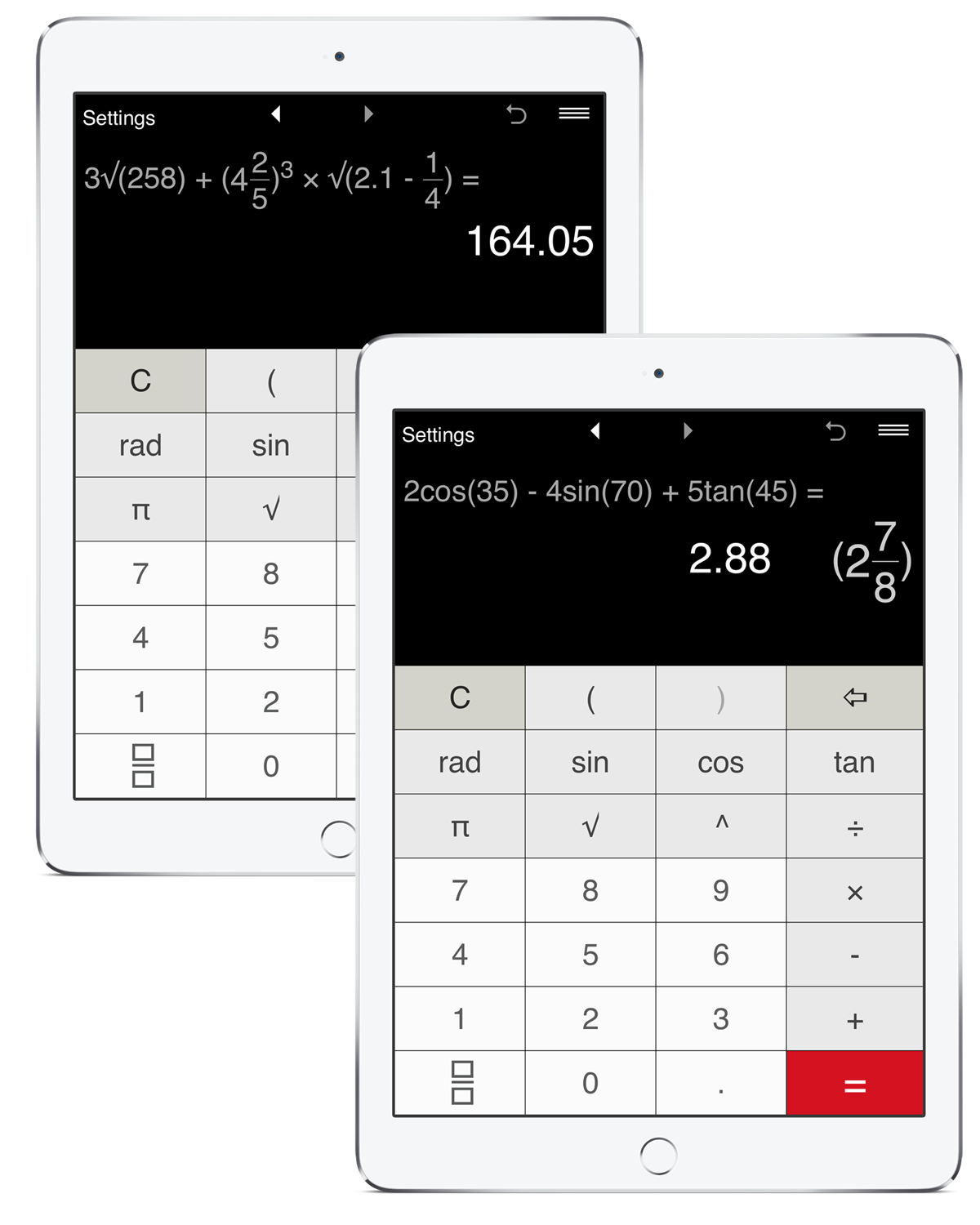## Fractions Pro Settings

• You may use the app in either portrait or landscape mode.
The calculator not only shows results in the decimal and fractional forms but also offers advanced rounding options for fractions and decimal numbers:
• In the Settings window you may easily set the accuracy of calculation by changing the number of decimal places from 0 to 8. So, you can set up the calculator to round decimal numbers to tenths, hundredths, thousandths etc. By default, the app shows 2 decimal places. If you need to round the result to the nearest whole number, just set the number of decimal places to '0'.
• Fractional results may be rounded to the nearest 1/2, 1/4, 1/8, 1/12, 1/16, 1/32, 1/64, 1/128, 1/256. If you do not need to round fractions, simply select "‑".
• You may customize the app appearance selecting one of the 7 color schemes.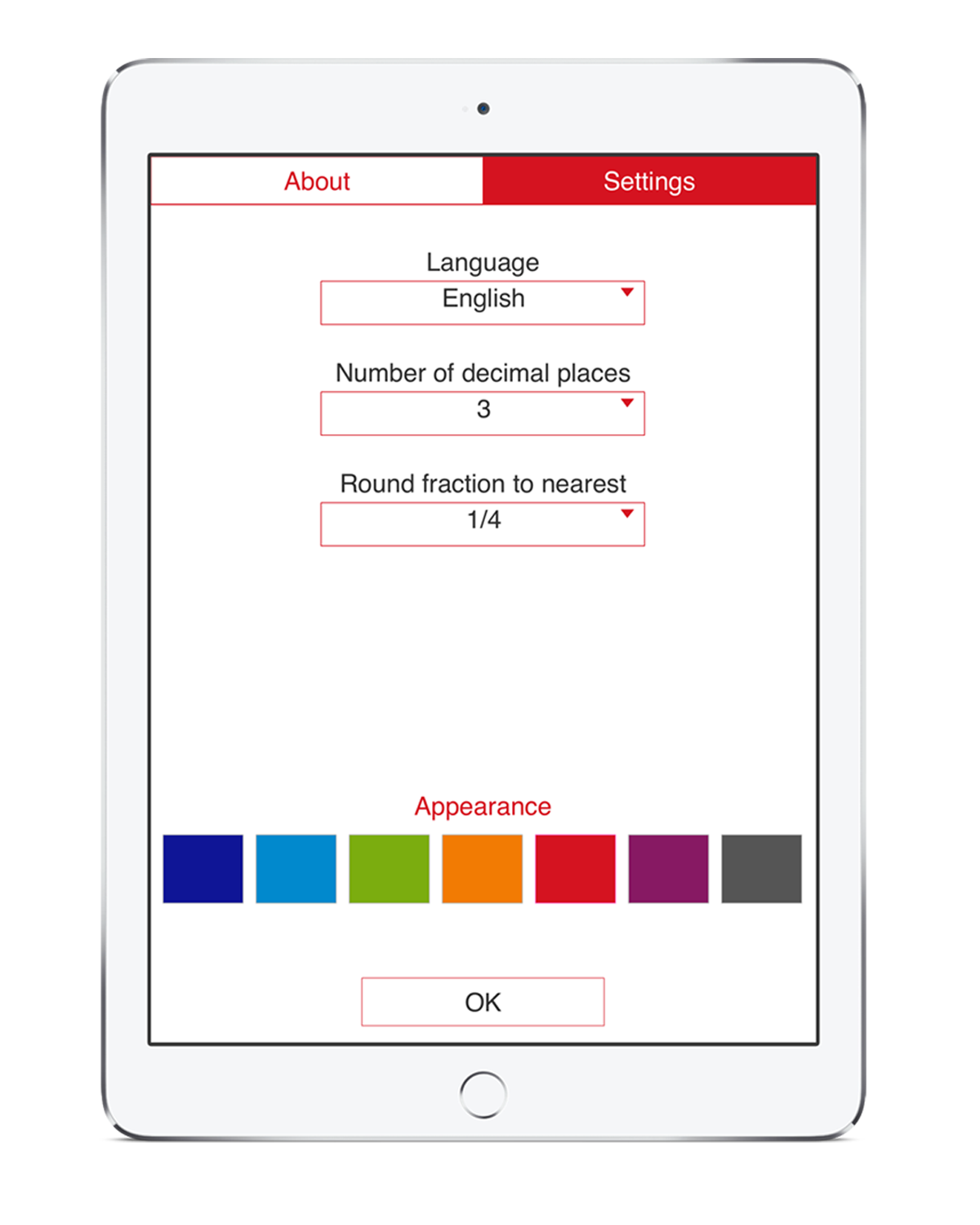### Special Functions• Intuitive interface for fraction input.• Back and Forward buttons to find and recall recent calculations.• 'Undo' for the Clear command.• History Tape to view and share calculations.• Share calculation results via email.